/

### Extreme Events – Specimen Question A.2.1(b) – Answer/Hints

Q. Do the statistics calculated in (a) appear to characterise a fat-tailed distribution if we adopt the null hypothesis that the log returns would otherwise be coming from a normal distribution and we use the limiting form of the distributions for these test statistics (i.e. the form ruling when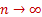,  where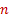is the number of observations)?

A (univariate) normal distribution is characterised by its mean and standard deviation, so the values of these two statistics cannot be used to differentiate between the normal distribution family and other distributional forms.

However, we can test for normality by reference to the observed skew and kurtosis of the sample. Ifis large and if the sample is drawn from a Normal distribution then the skew and (excess) kurtosis are approximately normally distributed with the following distributions, see also: MnConfidenceLevelSkewApproxIfNormal and MnConfidenceLevelKurtApproxIfNormal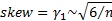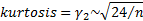Suppose we wish to reject the null hypothesis that the sample is coming from a normal distribution with a symmetric two-sided significance level of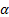(and we assume that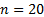is sufficiently large for the above approximations to apply), then we would reject the null hypothesis if the observed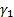and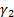are above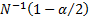or below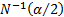where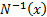is the (standardised) inverse normal distribution.can be obtained via the built-in Microsoft Excel worksheet function NORMSINV or via the equivalent Nematrian web function MnInverseNormal.

For example, if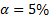then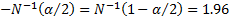. Thus at this level of significance, index A does not appear to be skewed, but does appear to be fat-tailed, since the observed value ofis 4.62 is significantly larger than 1.96.

Other tests for normality that might be used (including ones that can handle small samples and/or focus on just some parts of the overall distributional form) are described in TestsForNormality.